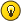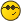# Tetration Forum

Full Version: [MSE] iterated sin using Besselfunction 1st kind
You're currently viewing a stripped down version of our content. View the full version with proper formatting.
This looks much promising!
In Nov 21 someone proposed to base the computation of iterated sin() on a matrix of Bessel-functions, diagonalize and get iterated sin() as composition of
$$a_1 \sin(x) + a_3 \sin(3x)+a_5 \sin(5x) +...$$

Just fiddled a bit with it, using Pari/GP, here some code

Code:
dpow(D,h) = for(k=1,#D,D[k]=D[k]^h); matdiagonal(D)    \\ power of a diagonalmatrix, given only the vector of diagonal entries MPow(e)=tmpM * dpow(tmpD,e) * tmpW[,1]          \\ note: the diagonalization-matrices are to be recalculated if dimension parameter is changed                                                                         \\ in the OP in MSE the user needs only column 1 of tmpW sinit(h,x='x)=my(coeffs); coeffs=2^(h-1)*MPow(h-1);sum(k=1,#coeffs,coeffs[k]*sin((2*k-1)*x))                                         \\ for further experiments it may be more sensible to keep the "coeffs" as global to better access them when comparing etc dim=16     M=matrix(dim,dim,r,c,besselj(2*r-1,2*c-1));        \\ define Besselmatrix of size dim x dim     tmpM=mateigen(M);tmpW=tmpM^-1;tmpD=diag(tmpW*M*tmpM)  \\ do diagonalization \\ some tests [ w1=sinit(3,1) ; s1=sin(sin(sin(1)));s1 - w1] %372 = [    0.678430477361] [    0.678430477361] [1.29470815679 E-23] [x05=sinit(1/2,1);  x1=sinit(1/2,x05) ; s1=sin(1); s1-x1] %374 = [    0.908708429656] [    0.841470984758] [    0.841470984808] [5.00517837623 E-11] \\ ********* Increasing matrixsize *************** \\ Using dim=32     \\ \\ ... don't forget to recompute matrices! \\ [ w1=sinit(3,1) ; s1=sin(sin(sin(1)));s1 - w1] %378 = [    0.678430477361] [    0.678430477361] [1.75726847276 E-50] [x05=sinit(1/2,1);  x1=sinit(1/2,x05) ; s1=sin(1); s1-x1] %380 = [    0.908708429743] [    0.841470984808] [    0.841470984808] [9.73370904483 E-14]

Looks very good, I think!

Gottfried
Excuse me Gottfried... but reading the title I can't resist saying that if any function comes out of iterated sin using Bessel functions then you should call it the Hell function, or call the code Devil.Pari/GP.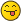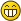(12/17/2022, 02:34 PM)MphLee Wrote: [ -> ]Excuse me Gottfried... but reading the title I can't resist saying that if any function comes out of iterated sin using Bessel functions then you should call it the Hell function, or call the code Devil.Pari/GP.Hmm, perhaps getting famous & holy if one eventually finds the sin()-root or perhaps, better: root-sin() ?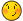(however, programming this in "Python", likely drives you out of paradise instead... isn't "Python" an objection-orientated language, btw.?)
Hey, Gottfried.

This is precisely the Fourier expansion of $$\sin^{\circ 1/2}(x)$$. It's well known this function is odd, and is also periodic. Thereby, when we expand the fourier expansion:

$\sin^{\circ 1/2}(x) = \sum_{k=1}^\infty a_k \sin(kx)\\$

Where:

$a_k = \frac{1}{2\pi}\int_{-\pi}^\pi \sin(kx)\sin^{\circ 1/2}(x)\,dx\\$

We can also deduce that $$a_{2k} = 0$$, because $$\sin^{\circ 1/2}(x)(\pi - x) = \sin^{\circ 1/2}(x+\pi/2)$$/// EDIT: SORRY I SCREWED UP THIS. I MEANT TO WRITE $$\sin^{\circ 1/2}(x)(\pi - x) = \sin^{\circ 1/2}(x)$$--which then eliminates even powers. I apoligize screwed that up a bit, lol. Which follows from basic trigonometric identities. Whereby we have a working:

$\sin^{\circ 1/2}(x) = \sum_{k=0}^\infty a_{2k+1} \sin(2k+1)x\\$

That being said, I've never seen this connection to the Bessel function. Cool find!!!! It makes sense though because one of the formulae for the bessel function of the first kind is an integral of nested sinusoids over $$[0,\pi]$$; and expanding these $$\sin^{\circ 1/2}(x)$$, will result in something very similar.

I also think, this provides a cool interchange of variables.

Write:

$\sin^{\circ 1/2}(x) = \frac{1}{\sqrt{\pi}}\int_0^\infty \frac{\vartheta(t,x)}{\sqrt{t}}\,dt\\$

Where:

$\vartheta(t,x) = \sum_{n=0}^\infty \sin^{\circ n+1}(x) \frac{(-t)^n}{n!}\\$

Then; then we can interchange these integrals, so that:

$a_{k}(1/2) = \frac{1}{\sqrt{\pi}} \int_0^\infty \sum_{n=0}^\infty a_{k}(n+1)\frac{(-t)^nt^{-1/2}}{n!} \,dt\\$

where:

$a_k(s) = \frac{1}{2\pi} \int_{-\pi}^\pi \sin^{\circ s}(x) \sin(kx)\,dx\\$

I've been aware of this formula for a long time, but I never saw the relation to Bessel before!

Also, note--I've never been able to prove this expansion of $$\sin^{\circ 1/2}(x)$$ is analytic. I only managed to show that it was  $$C^{\infty}$$ on $$(-\pi,\pi)$$. But I absolutely suspect it is analytic here. Maybe with closed forms of $$a_k$$ we'll have better luck!!!! I'd be really interested to see if this Fourier transform converges analytically, or only $$C^\infty$$. The sin iteration tends to be an odd ball out trying to get analycity out of. And showing uniform convergence of Fourier series in the complex plane is always a lot harder than it sounds (I think they've awarded like 4 fields medals for tiny step theorems on the convergence of Fourier series! lol!)
Hi James -

happy to see you saw this remark on the sinning-with-Bessel-functions. I've been already composing a PM to you, but cannot concentrate on one subject for enough time long... perhaps better next year. Since the main point of the PM should have been the pointing to this connection, it seems I can release now that half-ready draft :-)

Well, putting some comment here & there, perhaps a bit more, but I think more coherent stuff next year ... :-)

Kind regards -

Gottfried
I'm excited, Gottfried. I have a fair amount of time over the holidays, and then come January 10th, I'm homefree for 2-3 months. And I plan to work a lot on some of the ideas I've been mulling over--specifically cementing a lot of iteration theory using the Mellin transform! I'm busting out a lot of old notes which hand waived the crucial details, and rigorously justify them much more. Look forward to working with you!I'm excited to deep dive into the "Matrix method is the same as the integral method."

Regards, James

EDIT! Just a quick proof to show that the $$1,3,5,7,...$$ sequence in Gottfried's original post is nothing special.

$f(x) \,\,\text{is C^2 in}\,\,(-\pi,\pi)\\$

$\int_{-\pi}^\pi |f(x)|^2\,dx < \infty\\$

This is true for any iterate of $$\sin$$. Now:

$f(\pi - x) = f(x)\\$

What I think is more important here, is that $$f(x-\pi) = -f(x)$$. Which is what happens for every iterate of $$\sin$$. So let's work with the identity: $$f(x+\pi) = - f(x)$$.

Therefore:

$2\pi a_k = \int_{-\pi}^\pi f(x)sin(kx)\,dx = \int_0^\pi \left(f(x)\sin(kx)-(-1)^kf(x)\sin(kx)\right)\,dx$

Even values of $$k$$ are zero; odd values non-zero. Additionally, since:

$\sin^{\circ n}(-x) = -\sin^{\circ n}(x)\\$

We know:

$\int_{-\pi}^\pi f(x) \cos(kx)\,dx =0\\$

This is because this integrand is odd, across a symmetrical domain. Whereby, $$f(-x)\cos(-kx) = -f(x)\cos(kx)$$; and since we are integrating about zero over the same domain, the negative domain cancels the positive.

And so, when we call on the traditional Fourier series:

$f(x) = f(0) + \sum_{k=1}^\infty c_k \cos(kx) + a_k \sin(kx)\\$

We know, ABSOLUTELY, that $$c_k = 0$$ and $$a_{2k} = 0$$ and $$f(0) = 0$$. So that:

$f(x) = \sum_{k=0}^\infty a_{2k+1} \sin(2k+1)x\\$

Is the default expansion.

So the fact the MSE question pulled out this expansion is very much non-surprising, lol.

We want to use this to show that the integral transform coefficients I generate, are the same as the Bessel function coefficients this MSE question pulled out. I'd bet 50\$ they're the same expansion. But the matrix approach looks much more promising. Definitely, the Bessel matrices are quicker and more accurate--but they are the same thing mathematically as my integrals...

Hmm, perhaps a sweet new pseudonym... "St. RET. Cardinalis" ... let's see.